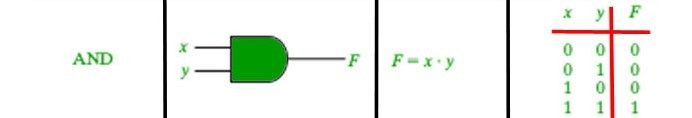# && operator in Java with Examples

&& is a type of Logical Operator and is read as “AND AND” or “Logical AND“. This operator is used to perform “logical AND” operation, i.e. the function similar to AND gate in digital electronics.One thing to keep in mind is the second condition is not evaluated if the first one is false, i.e. it has a short-circuiting effect. Used extensively to test for several conditions for making a decision.

Syntax:

```Condition1 && Condition2

// returns true if both the conditions are true.
```

Below is an example to demonstrate && operator:

Example:

 `// Java program to illustrate ` `// logical AND operator ` ` `  `import` `java.util.*; ` ` `  `public` `class` `operators { ` `    ``public` `static` `void` `main(String[] args) ` `    ``{ ` ` `  `        ``int` `num1 = ``10``; ` `        ``int` `num2 = ``20``; ` `        ``int` `num3 = ``30``; ` ` `  `        ``// find the largest number ` `        ``// using && operator ` `        ``if` `(num1 >= num2 && num1 >= num3) ` `            ``System.out.println( ` `                ``num1 ` `                ``+ ``" is the largest number."``); ` `        ``else` `if` `(num2 >= num1 && num2 >= num3) ` `            ``System.out.println( ` `                ``num2 ` `                ``+ ``" is the largest number."``); ` `        ``else` `            ``System.out.println( ` `                ``num3 ` `                ``+ ``" is the largest number."``); ` `    ``} ` `} `

Output:

```30 is the largest number.
```

Attention reader! Don’t stop learning now. Get hold of all the important DSA concepts with the DSA Self Paced Course at a student-friendly price and become industry ready.

My Personal Notes arrow_drop_upCheck out this Author's contributed articles.

If you like GeeksforGeeks and would like to contribute, you can also write an article using contribute.geeksforgeeks.org or mail your article to contribute@geeksforgeeks.org. See your article appearing on the GeeksforGeeks main page and help other Geeks.

Please Improve this article if you find anything incorrect by clicking on the "Improve Article" button below.

Article Tags :
Practice Tags :

Be the First to upvote.

Please write to us at contribute@geeksforgeeks.org to report any issue with the above content.